# JavaScript 是如何工作的：JavaScript 的内存模型

Fundebug经授权转载，版权归原作者所有。

// 声明一些变量并初始化它们
var a = 5;
let b = "xy";
const c = true;

// 分配新值
a = 6;
b = b + "z";
c = false; //  类型错误:不可对常量赋值

• JS 原始数据类型的变量声明和赋值
• JavaScript 内存模型：调用堆栈和堆
• JS 引用类型的变量声明和赋值
• let vs const

### JS 原始数据类型的变量声明和赋值

let myNumber = 23;

1. 为变量(myNumber)创建唯一标识符(identifier)。
2. 在内存中分配一个地址(在运行时分配)。
3. 将值 23 存储在分配的地址。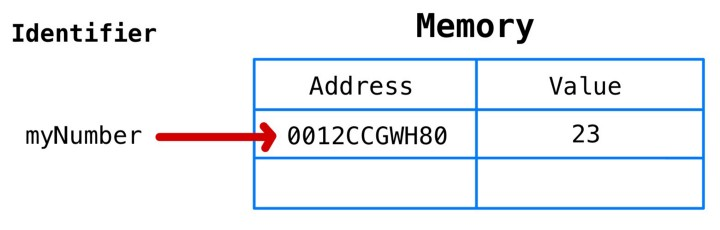let newVar = myNumber;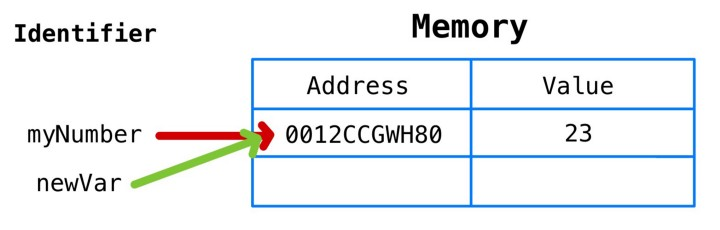myNumber = myNumber + 1;

myNumber的值肯定是 24。但是newVar的值是否也为 24 呢？，因为它们指向相同的内存地址？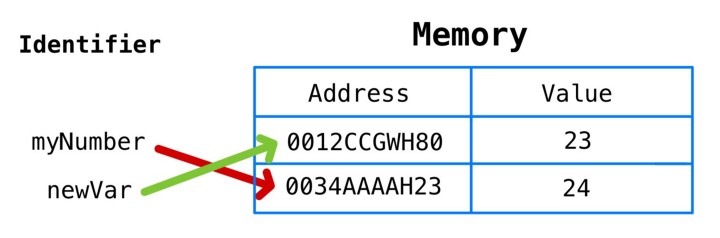let myString = "abc";
myString = myString + "d";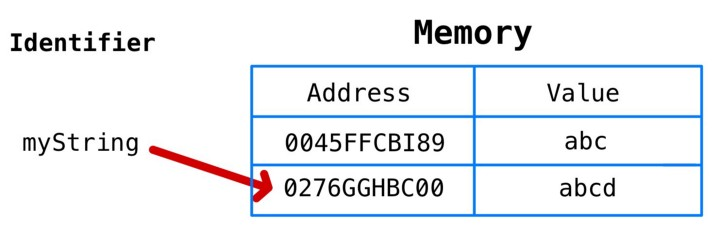### JavaScript 内存模型：调用堆栈和堆

JS 内存模型可以理解为有两个不同的区域:调用堆栈(call stack)堆(heap)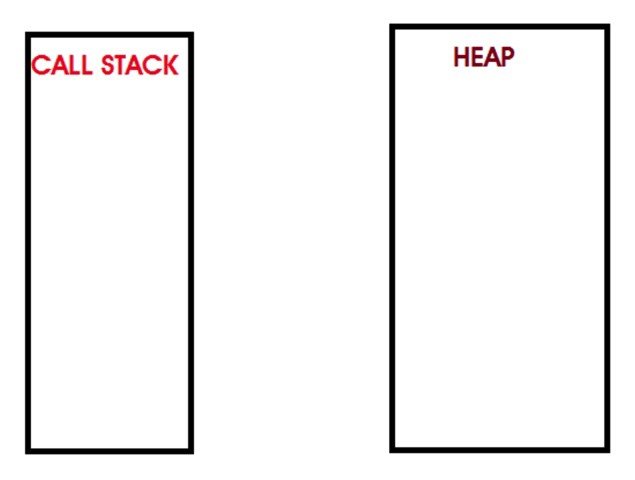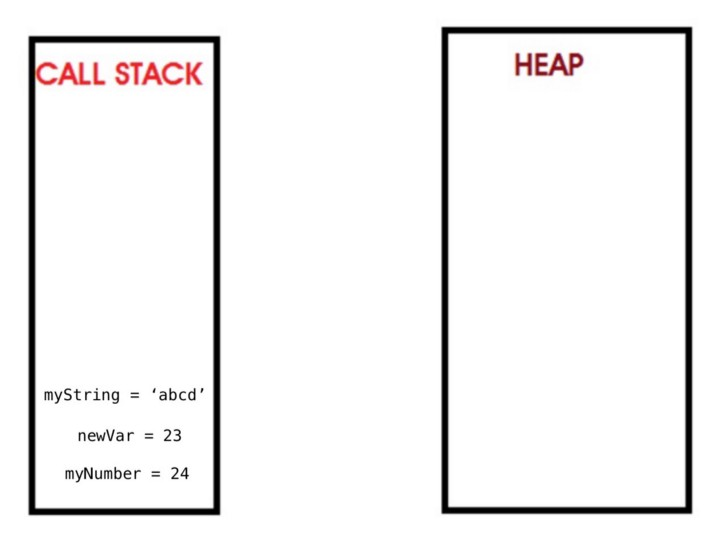### JS 引用类型的变量声明和赋值

let myArray = [];

1. 为变量创建唯一标识符（“myArray”）
2. 在内存中分配一个地址（将在运行时分配）
3. 存储在堆上分配的内存地址的值（将在运行时分配）
4. 堆上的内存地址存储分配的值（空数组[]）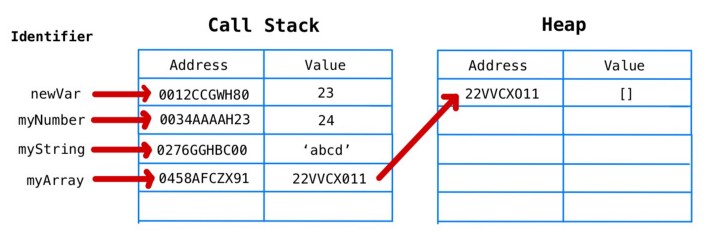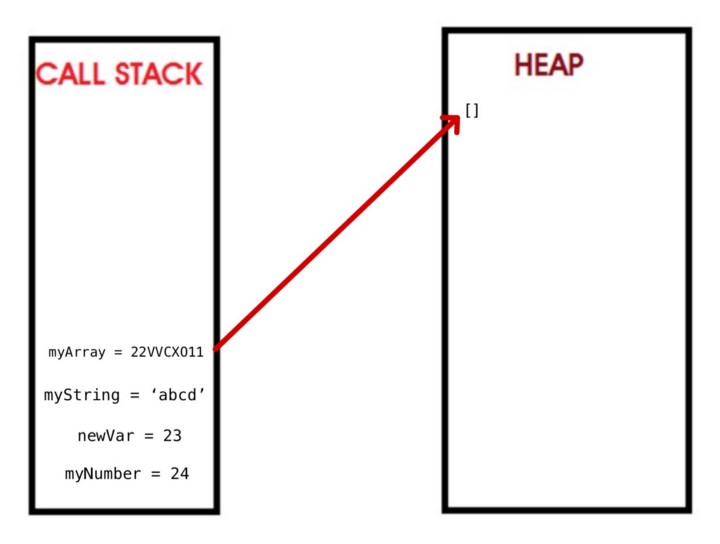myArray.push("first");
myArray.push("second");
myArray.push("third");
myArray.push("fourth");
myArray.pop();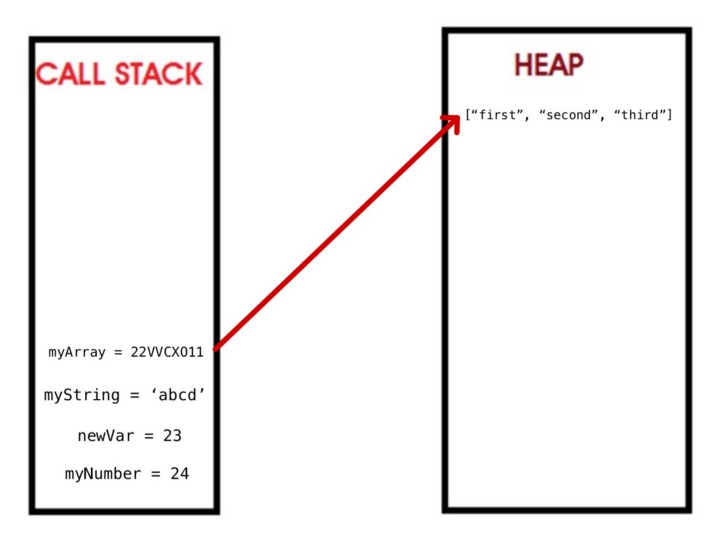### let vs const

let sum = 0;
sum = 1 + 2 + 3 + 4 + 5;
let numbers = [];
numbers.push(1);
numbers.push(2);
numbers.push(3);
numbers.push(4);
numbers.push(5);

const importantID = 489;
importantID = 100; // 类型错误:赋值给常量变量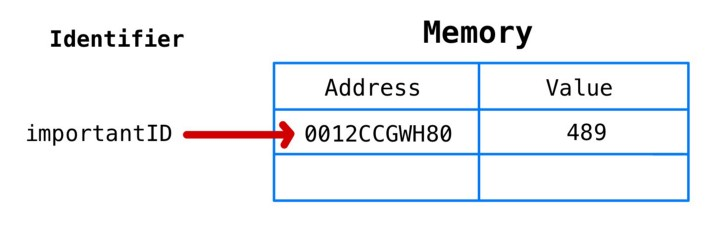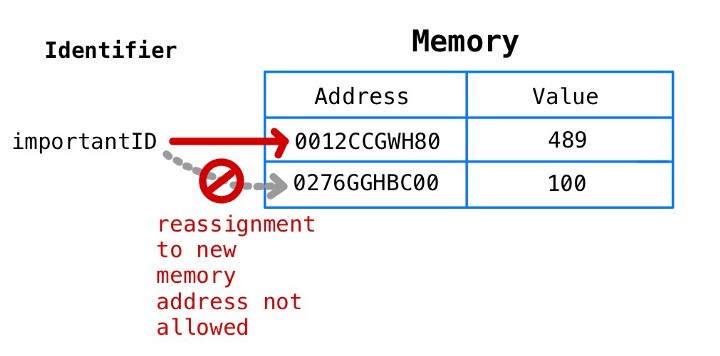const myArray = [];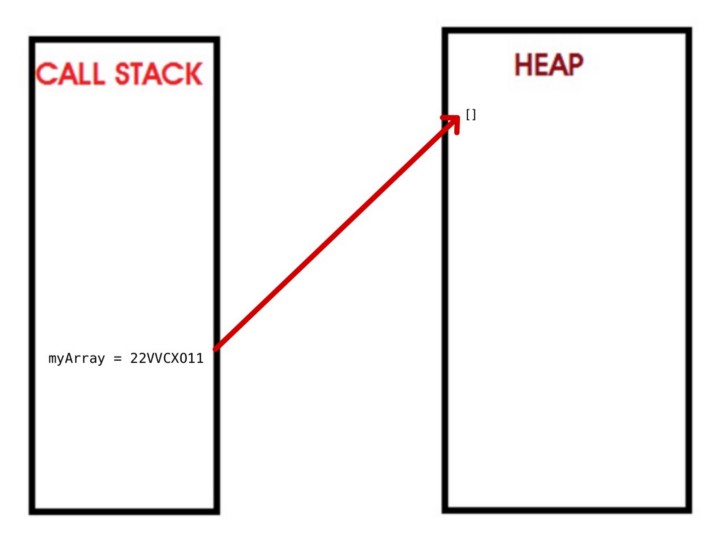myArray.push(1);
myArray.push(2);
myArray.push(3);
myArray.push(4);
myArray.push(5);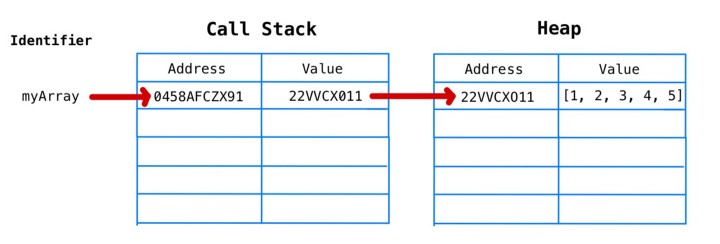myArray 仍然等于 0458AFCZX91，它的值是另一个内存地址22VVCX011，它在堆上有一个数组的值。

myArray = 3;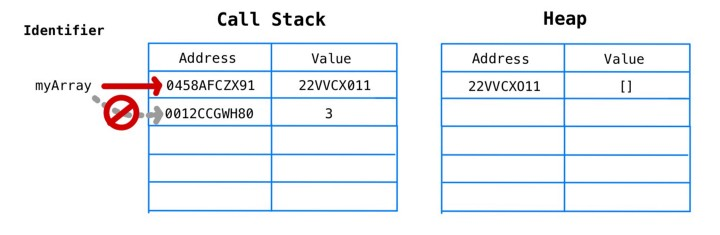myArray = ["a"];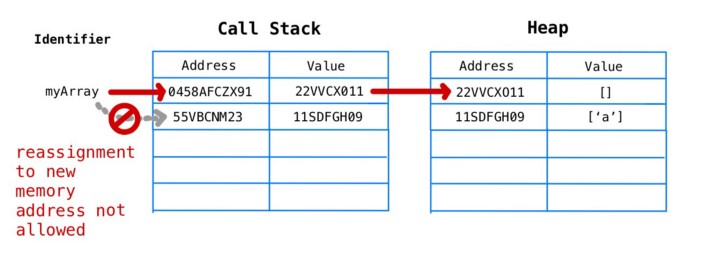const myObj = {};
myObj["newKey"] = "someValue"; // 这不会抛出错误

### 为什么这些知识对我们有用呢

JavaScript 是世界上排名第一的编程语言（根据 GitHub 和 Stack Overflow 的年度开发人员调查）。 掌握并成为“JS 忍者”是我们所有人都渴望成为的人。

• 先发制人地限制未来的 bug。
• 使用 const 声明的变量必须在声明时初始化，这迫使程序员经常在范围方面更仔细地放置它们。这最终会导致更好的内存管理和性能。
• 要通过代码与任何可能遇到它的人交流，哪些变量是不可变的(就 JS 而言)，哪些变量可以重新分配。

### 关于Fundebug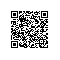使用钉钉扫一扫加入圈子
+ 订阅